# Fan Wiki

Fan:
A fan is a turbo-machine intend for the continuous displacement of air or other gases up to a pressure ratio of pout / pin of 1.3.
The classification of fans refers to the kind of installation and the operating mode.

Type of fan:
Fans are to classify into centrifugal-, axial- and diagonal-fans.

Characteristics of fans

Flow coefficient
The flow coefficient is the ratio between the real and the theoretically flow rate (product of the circular area of the impeller and the circumferential velocity).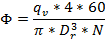Φ = Flow coefficient
qv = Flow rate [m³/s]
Dr = Impeller outer diameter [m]
N = Rotational speed [1/min]

Pressure coefficient
The pressure coefficient is the ratio between the pump head generated by the impeller and the ram pressure of the circumferential velocity.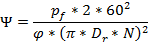ψ = Pressure coefficient
ρ = Density [kg/m³]
pf = Fan pressure [Pa]
Dr = Impeller outer diameter [m]
N = Rotational speed [1/min]

Power density
The power density is the product of the flow coefficient and the pressure coefficient.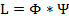L = Power density
Φ = Flow coefficient
ψ = Pressure coefficient

Fan efficiency
The fan efficiency describes the ratio of the gas power output and the electrical power input.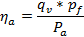ηa = Fan efficiency
qv = Flow rate [m³/s]
pf = Fan pressure [Pa]
Pa = Shaft power [W]

Performance coefficient
The performance coefficient is a dimensionless number to characterize the shaft power of a fan.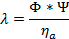λ = Performance coefficient
Φ = Flow coefficient
ψ = Pressure coefficient
ηa = Fan efficiency

Diameter coefficient
The diameter coefficient is a dimensionless number to describe the outside diameter of the impeller compared with a fan with ψ = 1 and φ = 1.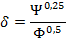δ = Diameter coefficient
Φ = Flow coefficient
ψ = Pressure coefficient

Required power of the fan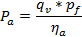Pa = Shaft power [W]
qv = Flow rate [m³/s]
pf = Fan pressure [Pa]
ηa = Fan efficiency

Required power of the motor

Pe  = Required motor power [kW]
Pa = Required shaft power [kW]

ηG  = Efficiency of the power transmission components
ηo  = Motor efficiency

Efficiencies of the power transmission components:

• rigid coupling: 1,00
• V-belt, single belt: 0,93 ... 0,95
• V-belt, multiple belt: 0,90 ... 0,93
• Ribbed v-belt: 0,97
• Flat belt: 0,96 ... 0,99
• Toothed v-belt: 0,98 ... 0,99

Average motor efficiency:

Motor power [kW] Efficiency Motor power [kW] Efficiency
1,1
0,820
15,0
0,920
90
0,948
1,5
0,830
18,5
0,915
110
0,948
2,2
0,859
22,0
0,923
132
0,955
3,0
0,865
30,0
0,923
160
0,956
4,0
0,870
37,0
0,927
5,5
0,880
45,0
0,930
7,5
0,899
55,0
0,939
11,0
0,900
75,0
0,940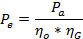Conversion of the performance characteristics for pole-changing motors

Number of poles
Volume flow
Pressure
Power
2 / 4
qv1 / qv2 = 1 / 2
pf1 / pf2 = 1 / 4
Pa1 / Pa2 = 1 / 8
4 / 8
qv1 / qv2 = 1 / 2
pf1 / pf2 = 1 / 4
Pa1 / Pa2 = 1 / 8
6 / 12
qv1 / qv2 = 1 / 2
pf1 / pf2 = 1 / 4
Pa1 / Pa2 = 1 / 8
4 / 6
qv1 / qv2 = 1 / 1,5
pf1 / pf2 = 1 / 2,25
Pa1 / Pa2 = 1 / 3,375
8 / 12
qv1 / qv2 = 1 / 1,5
pf1 / pf2 = 1 / 2,25
Pa1 / Pa2 = 1 / 3,375
6 / 8
qv1 / qv2 = 1 / 1,33
pf1 / pf2 = 1 / 1,778
Pa1 / Pa2 = 1 / 2,37

Conversion of the flow rate for different rotation speed
Modification of the volume flow as a function of the rotation speed
The volume flow is changing proportional to the rotation speed.

qv1 = Volume flow operating point 1 [m³/s]
qv2 = Volume flow operating point 2 [m³/s]
N1  = Rotation speed operating point 1 [1/min]
N2 = Rotation speed operating point 2 [1/min]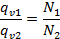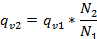Modification of fan pressure as a function of rotational speed
The pressure increase is changing with the square of the rotation speed.

pf1 = Fan pressure increase at operating point 1 [Pa]
pf2 = Fan pressure increase at operating point 2 [Pa]
N1 = Rotational speed at operating point 1 [1/min]
N2 = Rotation speed at operating point 2 [1/min]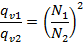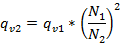Modification of shaft pover as a function of rotational speed
The power requirement is changing with the third power of the rotation speed.

Pa1 = Required shaft power at operating point 1 [kW]
Pa2 = Required shaft power at operating point 2 [kW]
N1 = Rotational speed at operating point 1 [1/min]
N2 = Rotation speed at operating point 2 [1/min]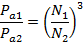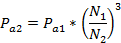Conversion of flow rate to another impeller outer diameter
Modification of the volume flow as a function of the impeller diameter
The volume flow is changing with the third power of the impeller diameter.

qv1 = Flow rate [m³/s]             with impeller diameter Dr1 [m]
qv1 = Flow rate [m³/s]             with impeller diameter Dr2 [m]
Dr1  = Impeller outer diameter 1 [m]
Dr2 = Impeller outer diameter 2 [m]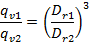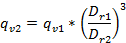Modification of fan pressure as a function of the impeller outer diameter
The pressure increase is changing with the second power of the impeller diameter.

pf1 = fan pressure [Pa] with Impeller outer diameter Dr1
pf2 = fan pressure [Pa] with Impeller outer diameter Dr2
Dr1  = Impeller outer diameter 1 [m]
Dr2 = Impeller outer diameter 2 [m]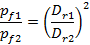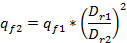Modification of shaft power as a function of the impeller outer diameter:
The power requirement is changing with the fifth power of the impeller diameter.

Pa1 = Required shaft power [kW] at Impeller outer diameter Dr1
Pa2 = Required shaft power [kW] at Impeller outer diameter Dr2
Dr1  = Impeller outer diameter 1 [m]
Dr2 = Impeller outer diameter 2 [m]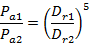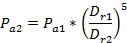Conversion of the fan data for a modified air density and air temperature:
The performance data of air in a catalogue are usually valid for a density of p = 1,2 kg/m³ with an air temperature of 20°C and a pressure of 101300 Pa (standard condition). For a modified air density the generated fan pressure pf and the required shaft power Pa are changing proportional with density. The flow rate stays constant.

Air density as a function of the temperature

ρ = Air density [kg/m³]
pa = Air pressure [Pa] – Standard condition 101.300 Pa
R = Gas constant [J/(kg*K] – for air 287 J/kg*K
t = Reference temperature [°C]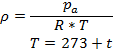Modification of fan pressure with a change of the air density

pf1 = fan pressure [Pa] at density 1 [kg/m³]
pf2 = fan pressure [Pa] at density 2 [kg/m³]
ρ1 = Air density 1 [kg/m³]
ρ2 = Air density 2 [kg/m³]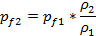Modification of shaft power with a change of the air density

Pa1 = Required shaft power [kW] at density 1 [kg/m³]
Pa2 = Required shaft power [kW] at density 2 [kg/m³]
ρ1 = Air density 1 [kg/m³]
ρ2 = Air density 2 [kg/m³]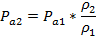Modification of fan pressure with a change of the air temperature

pf1 = fan pressure [Pa] at temperature 1 [°C]
pf2 = fan pressure [Pa] at temperature 2 [°C]
T1 = Air temperature 1 [K]
T2 = Air temperature 2 [K]
t = Reference temperature [°C]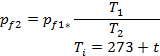Modification of shaft power with a change of the air temperature

Pa1 = Required shaft power [kW] at temperature 1 [°C]
Pa2 = Required shaft power [kW] at temperature 2 [°C]
T1 = Air temperature 1 [K]
T2 = Air temperature 2 [K]
t = Reference temperature [°C]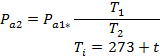Sound levels of fans

Estimation of the total sound power of fans
The calculated total sound power with the formula below is only a kind of estimation. This value is approximately realistic when the fan is working in the best possible operating point. I. e. at the maximum efficiency. The detailed values are to find in the data sheet of the manufacturer.

Sound levels of fans

Estimation of the total sound power of fans
The calculated total sound power with the formula below is only a kind of estimation. This value is approximately realistic when the fan is working in the best possible operating point. I. e. at the maximum efficiency. The detailed values are to find in the data sheet of the manufacturer.

LW = Total sound power level [dB] ± 4 dB
qv = flow rate [m³/s]
pf = Fan pressure [Pa]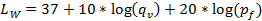Determination of the octave power level
The following values at the single frequencies are to subtract from the total sound power level to determine the frequency related octave power level.
Type of fan-level difference [dB] at octave-frequency

Hz
63
125
250
500
1000
2000
4000
8000
Typ 1
2
7
12
17
22
27
32
37
Typ 2
9
8
7
12
17
22
26
31
Typ 3
9
8
7
7
8
10
14
18

Typ 1 = Centrifugal fan / drum fan with forward curved blades
Typ 2 = Centrifugal heavy-duty fan with backward curved blades
Typ 3 = Axial fan

Influence of the rotation speed on the sound level
Modification of the sound level with a change of the rotational speed
ΔL = Change in sound level [dB]
N = New rotational speed [1/min]
N0= Nominal rotational speed [1/min]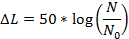Rotation frequency of a fan (Main interfering frequency)
fD = Rotational frequency [Hz]
N = Rotational speed [1/min]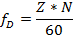Manufacturer of heavy industrial fans with production and headquarters in Germany. Individual and tailor-made exhaust air solutions for industry.

The extensive know-how of the traditional company DLK Ventilatoren guarantees continuous innovation.

## Contact

DLK Ventilatoren GmbH
Ziegeleistraße 18
74214 Schöntal-Berlichingen
Phone: +49 7943 9102 0
Fax: +49 7943 9102 10
Email: info@dlk.com

changing Language

Sprache wird geändert